# Turbine Meter Totalisation Check

## Form

Form: K134 – Liquid Totalisation – Pulse Output Meter

Form Reference: C052

Calculation Details

## Purpose

The purpose of this test is to check the totals calculated by the flow computer are correct by injecting a number of pulses to generate totals.

## Test Equipment

A dual pulse simulator is selected for the test equipment. Other options may include a signal generator and timer/counter.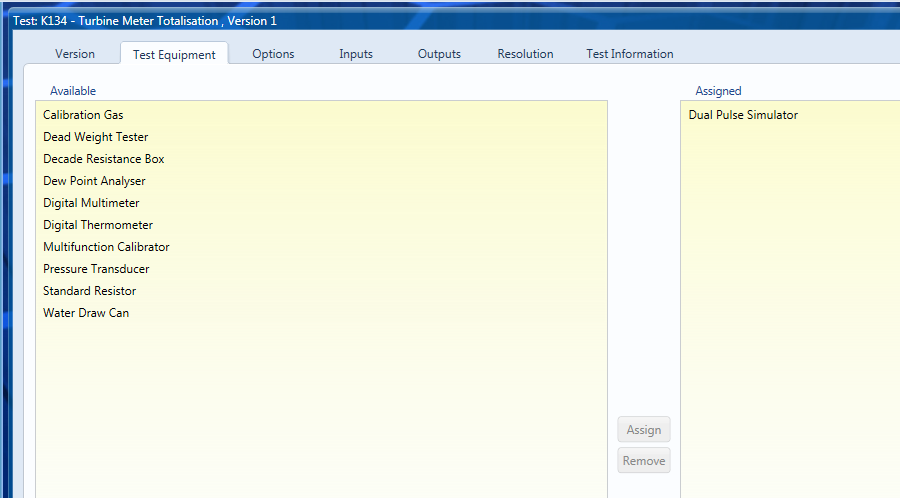## Options

In this example we are checking a turbine meter where the output volume is proportional to the number of pulses injected. The total can also be generated by injecting a frequency over set period time, this can be set within the Method option group. We are not correcting for Ctsm and Cpsm assuming the K-Factor is determined at the operating pressure and temperature. We are calculating both the volume at observed conditions, the standard volume and the mass. The line and standard densities are set to be user entered but density referral calculations are available if you wish to use them.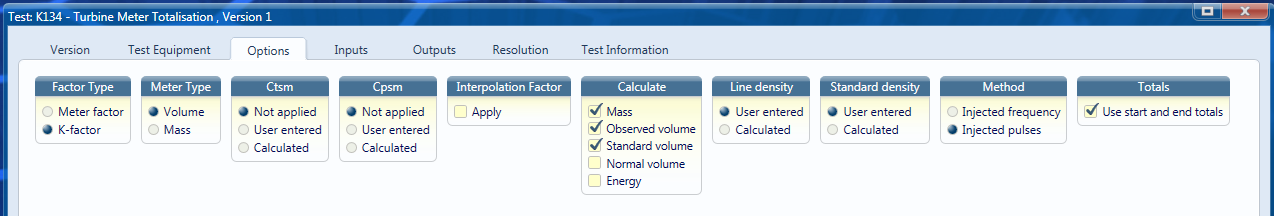## Inputs

All inputs are set to be entered at the time of the test. Inputs can be set to fixed values or user entry with default for user convenience.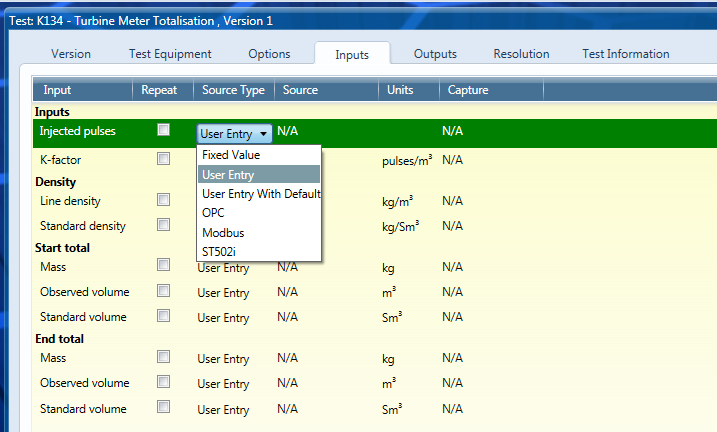## Outputs

The calculated totals (from the pulse input) are validated against the difference in the start and end totals. To do this select Calculated Value as the Source Type. In the Source drop down the relevant total will be available for selection.

The Error Mode will be percentage of calculated value. A typical tolerance for this check will be 0.001 % of calculated value.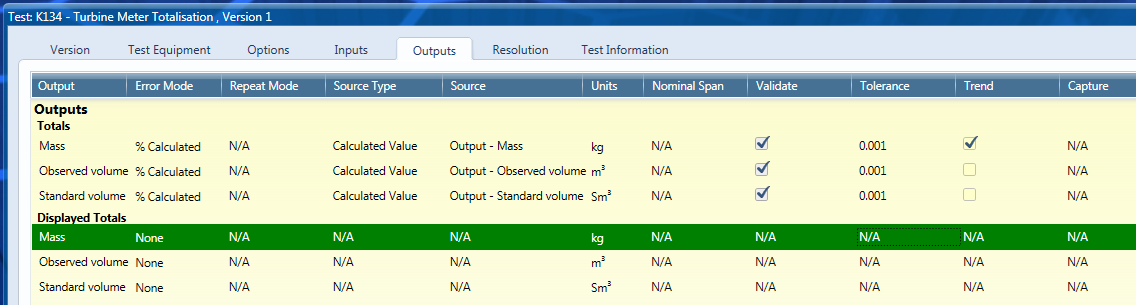The displayed totals (calculated from the start and end totals inputs) can be hidden by clicking Manage from the Configuration ribbon. Here the outputs displayed can be chosen by the user.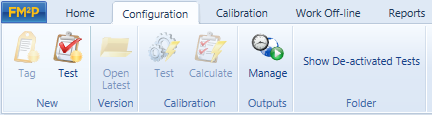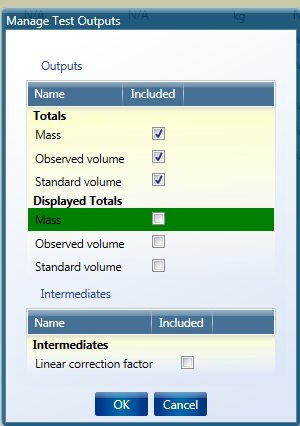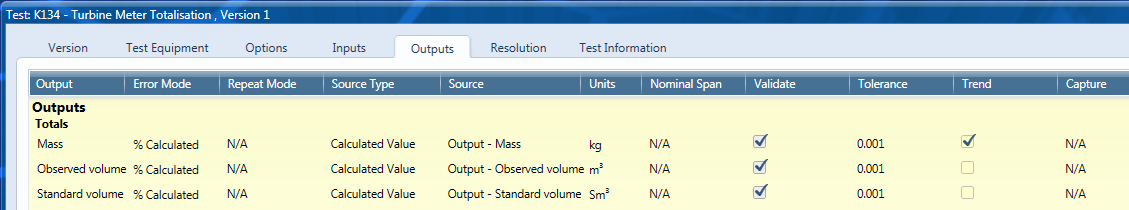Back